# Quant Quiz for IBPS SO and CLERK MAINS 2018 | 2nd January 2019

Quant Quiz to improve your Quantitative Aptitude & Data Interpretation. For upcoming exams like Bank, SSC, Railway, UPSC, UPSSSC, CDS, UPTET, KVS, DSSSB and other Government exams.

Q1. A man can row a distance of 2 km upstream in 15 minutes and returns the same distance in 10 minutes. How much time will he take to row the same distance upstream if due to a tide the speed of the current gets doubled ?(a) 25 minutes?

(b) 45 minutes

(c) 20 minutes

(d) Can’t be determined

(e) None of these

Q2. Two trains have respective lengths as 230 m and 190 m. They cross each other completely in 21 s, if they are travelling in the opposite direction and in 42 s, if they are traveling in the same direction. Find the ratio of the speed of the two trains?

(a) 3 : 1

(b) 4 : 1

(c) 3 : 2

(d) Can’t be determined

(e) None of these

Q3. A person has 12 friends of whom 8 are relatives. In how many ways can he invite 7 friends such that at least 5 of them may be relatives ?

(a) 284

(b) 384

(c) 456

(d) 512

(e) None of these

Q4. The diameter of a road-roller is 42 cm and its length is 100 cm. It takes 400 complete revolutions moving once over to level the stretch of the road. If the cost of leveling is Rs. 100 per m2 then the total cost of leveling works out to?

(a) Rs. 52,800

(b) Rs. 5,280

(c) Rs. 5,28,000

(d) Rs. 528

(e) None of these

Q5. The biggest possible cube is taken out of a right solid cylinder of radius 15 cm and height 20 cm respectively. What will be the volume (in cm3) of the cube ?

(a) 375

(b) 800

(c)

(d) Can’t be determined

(e) None of these

Directions (Q6 –Q10): The bar graph given below shows the sales of books (in thousand) from six branches of a publishing company during two consecutive years 2010 and 2011.

Sales of books (in thousand) from six branches B1, B2, B3, B4, B5, B6 of a publishing company in 2010 and 2011.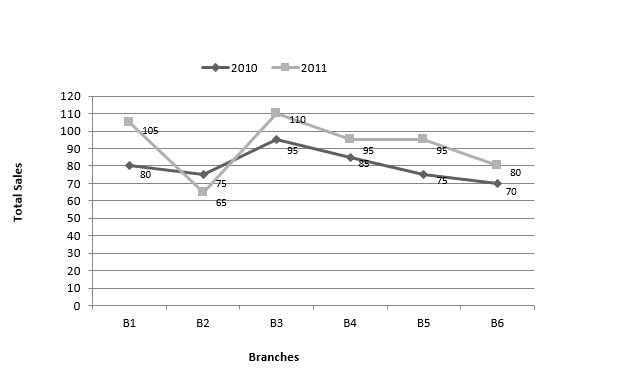Q6. What is the ratio of total sales of B1, B4 and B6 to the total sale of B2 in 2011, B3 in 2010 and B5 in 2010?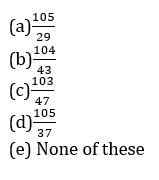Q7. If total sale of B2 is increased by 20%  in 2010 and sale of B1, B3 and B4 are decreased by 10%, 15% and 5% respectively in 2010 then, what will be the new average of total sales of all the branches for both years?

(a) 170

(b) 178
(c) 182

(d) 180

(e) 172

Q8. If the number of articles sold by branch B5 in 2010 and 2011 are 3 and 5 respectively and number of articles sold by branch B6 in 2010 and 2011 is 5 and 4 respectively then what will be the approximate difference between total profit per article of branch B5 and B6 for both years ? Profit of B5 in 2010 and 2011 is 10% and 15% respectively and profit for B6 in 2010 and 2011 is 12% and 20% respectively?

(a) 0.06

(b) 0.08
(c) 0.1

(d) 0.01

(e) 0.05

Q9.  Sale of branch B3 in 2010 and 2011 is increased by 20% and  40% respectively and sale of B2 in 2010 and sale of B6 in 2011 is increased by 4% and 5% respectively.  What will be the ratio of total sale of B2 and B6 in both years to the sum of  sale of B3 in both years and sale of B1 in 2010?Q10. Sale of branch B3 in 2012 is 80% of the  sale of B3 in both year and  sale of branch B1 in 2009 is 90% of sale of branch B1 in 2010. By what % (approx.) difference between total sale of B3 in 2012 and total sale of B1 in 2009 is less than total sale of all branches in both the years taken together?

(a) 80%

(b) 91%
(c) 75

(d) 12%

(e) None of these

# Solutions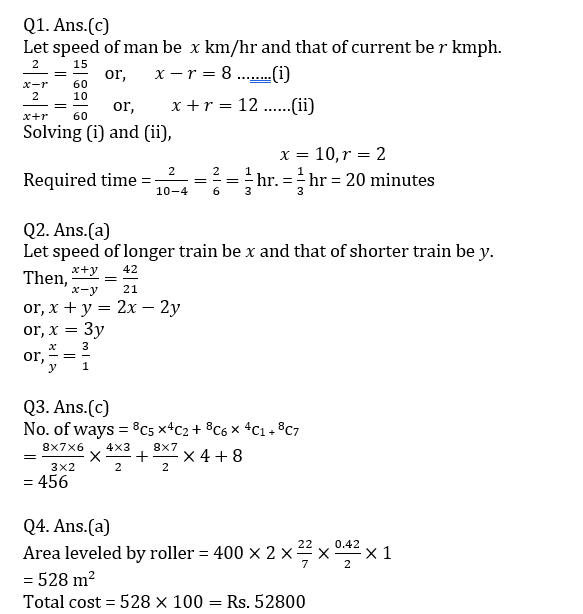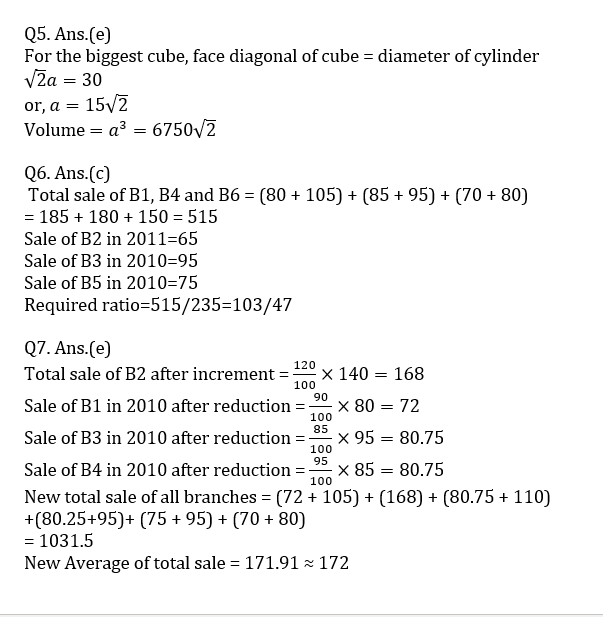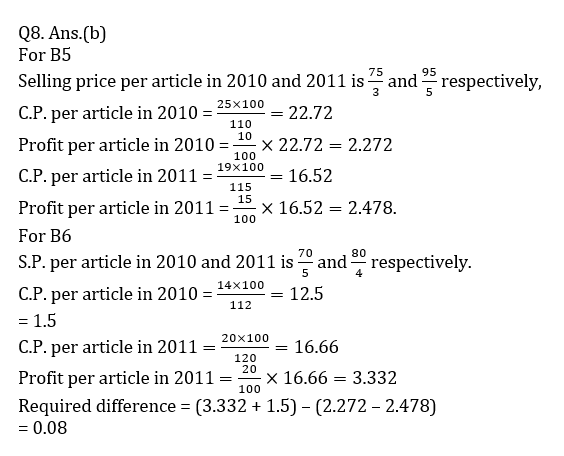## WhatsApp Group Join here

Mail us at : ambitiousbaba1@gmail.com